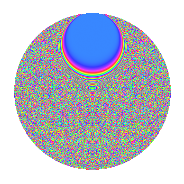# Properties

 Label 2006.2.rLevel 2006 Weight 2 Character orbit r Rep. character $$\chi_{2006}(9,\cdot)$$ Character field $$\Q(\zeta_{232})$$ Dimension 10080 Sturm bound 540

# Related objects

## Defining parameters

 Level: $$N$$ = $$2006 = 2 \cdot 17 \cdot 59$$ Weight: $$k$$ = $$2$$ Character orbit: $$[\chi]$$ = 2006.r (of order $$232$$ and degree $$112$$) Character conductor: $$\operatorname{cond}(\chi)$$ = $$1003$$ Character field: $$\Q(\zeta_{232})$$ Sturm bound: $$540$$

## Dimensions

The following table gives the dimensions of various subspaces of $$M_{2}(2006, [\chi])$$.

Total New Old
Modular forms 30688 10080 20608
Cusp forms 29792 10080 19712
Eisenstein series 896 0 896

## Trace form

 $$10080q + 8q^{9} + O(q^{10})$$ $$10080q + 8q^{9} + 8q^{11} - 8q^{12} - 16q^{14} - 24q^{15} + 360q^{16} + 24q^{18} - 16q^{19} - 16q^{22} + 32q^{23} - 8q^{24} - 24q^{27} + 16q^{29} - 32q^{31} - 32q^{33} + 32q^{34} - 32q^{35} + 8q^{36} - 16q^{37} + 32q^{39} - 16q^{41} + 32q^{42} + 8q^{43} + 16q^{44} - 40q^{45} - 32q^{46} - 48q^{49} - 488q^{50} + 16q^{52} + 16q^{53} + 804q^{54} - 16q^{57} + 4q^{59} - 672q^{60} - 16q^{61} - 24q^{63} + 64q^{65} + 24q^{66} - 448q^{67} + 64q^{69} + 416q^{71} + 64q^{73} - 32q^{75} + 16q^{76} - 32q^{77} + 16q^{79} + 24q^{82} - 8q^{83} - 96q^{85} - 16q^{87} + 24q^{88} + 32q^{91} + 16q^{92} - 128q^{93} - 32q^{94} + 24q^{97} - 396q^{99} + O(q^{100})$$

## Decomposition of $$S_{2}^{\mathrm{new}}(2006, [\chi])$$ into irreducible Hecke orbits

The newforms in this space have not yet been added to the LMFDB.

## Decomposition of $$S_{2}^{\mathrm{old}}(2006, [\chi])$$ into lower level spaces

$$S_{2}^{\mathrm{old}}(2006, [\chi]) \cong$$ $$S_{2}^{\mathrm{new}}(1003, [\chi])$$$$^{\oplus 2}$$